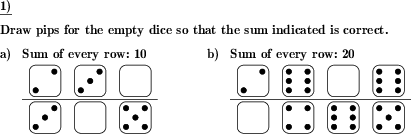Custom math worksheets at your fingertips# Details for problem "Draw dice pips for correct sum"

Quickname: 5491

Elementary School, Primary School.

## Summary

First steps to subtraction skills: Add dice pips so that sum of multiple dice is correct.

## Example## Description

This problem can be used to introduce the complement method for subtraction.

Rows of dice faces are shown. One of the dices' faces is empty. Pips have to be drawn for this dice so that the total sum of all the dice in the row equals a given value.

The number of problems and the number of rows for a problem can be selected. The Sum that all dice face have to add up to can be specified in the following way:

• ten for two or three dice, 20 for four dice
• ten for two dice, 15 for three and 20 for four dice
• a random value

Every set of rows for a problem targets the same sum. The rows of dice are separated by lines. This may be misleading in some situations or for some audiences, it is advisable to select only a single row per problem in this case.

Download free printable worksheets for this math problem here. The worksheet contains the problems only, the solution sheet includes the answers. Just click on the respective link.

•Worksheet 1Solution sheet with answers
•Worksheet 2Solution sheet with answers
•Worksheet 3Solution sheet with answers

If you can not see the solution sheets for download, they may be filtered out by an ad blocker that you may have installed. If this is the case, please allow ads for this page and reload the page. The solution sheets will then reappear.

• Do these sample worksheets do not really fit?
• Do you need more math worksheets, with a different level of difficulty?
• Would you like to combine different problems on a worksheet and adjust them to your needs?
• As a teacher, you can put together your own worksheets using the automatically generated math problems provided.
With a free initial credit, you can start creating your own math worksheets in a few minutes.

You can try it for free! Register here, to create custom worksheets now!

## Customization options for this problem

Parameter
Possible values
Number of problems
1, 2, 3, 4, 5, 6
Number of rows per problem
1, 2, 3
Number of dice
2-4, 2, 3, 4
Sum
10 or 20, 10, 15 or 20, random

## Similar problems

Remark
Description
Just count the objects
A series of different symbols is shown. Count each of the symbols.
Graphical approach to subtraction in the range from one to ten
A series of marbles represents a subtraction term - empty spaces to be filled in term.